# Key Financial Indicators Key Financial Indicators Measures of

• Slides: 13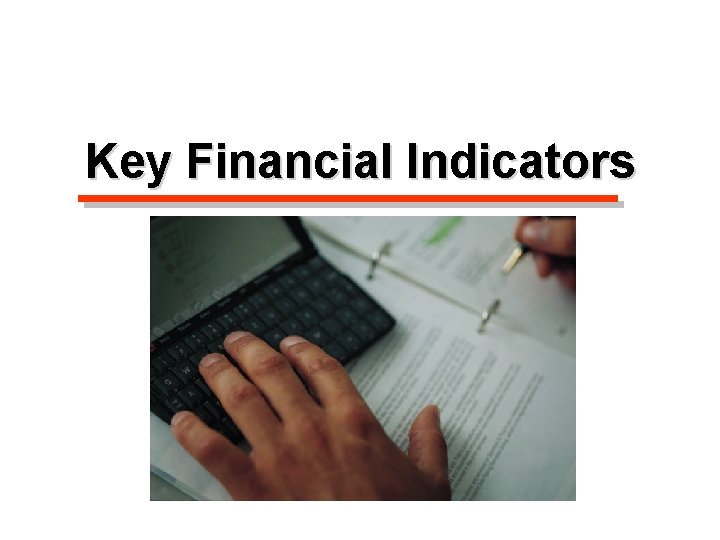Key Financial Indicators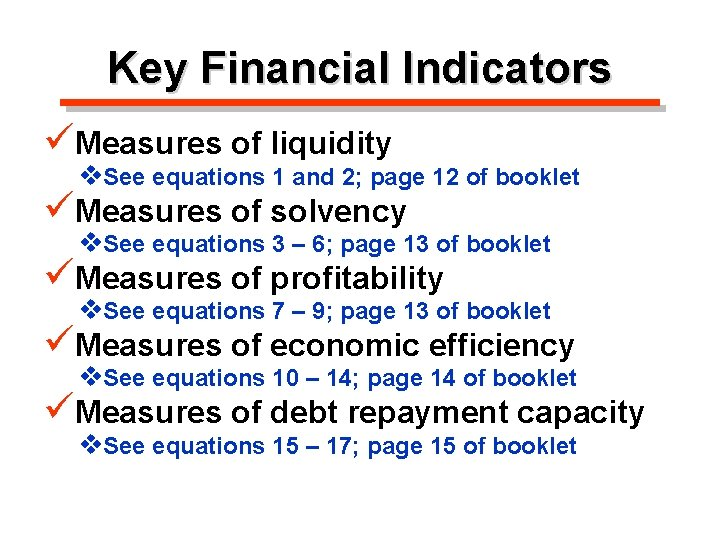Key Financial Indicators üMeasures of liquidity v. See equations 1 and 2; page 12 of booklet üMeasures of solvency v. See equations 3 – 6; page 13 of booklet üMeasures of profitability v. See equations 7 – 9; page 13 of booklet üMeasures of economic efficiency v. See equations 10 – 14; page 14 of booklet üMeasures of debt repayment capacity v. See equations 15 – 17; page 15 of booklet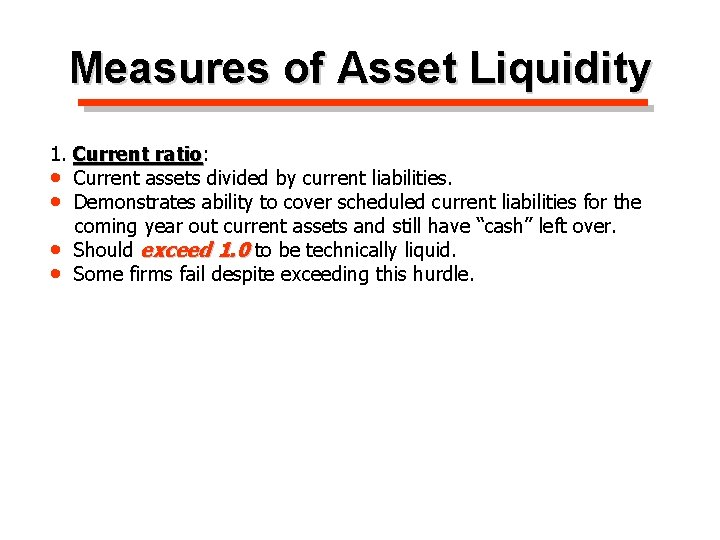Measures of Asset Liquidity 1. Current ratio: ratio • Current assets divided by current liabilities. • Demonstrates ability to cover scheduled current liabilities for the coming year out current assets and still have “cash” left over. • Should exceed 1. 0 to be technically liquid. • Some firms fail despite exceeding this hurdle.Measures of Asset Liquidity 1. Current ratio: ratio • Current assets divided by current liabilities. • Demonstrates ability to cover scheduled current liabilities for the coming year out current assets and still have “cash” left over. • Should exceed 1. 0 to be technically liquid. • Some firms fail despite exceeding this hurdle. 2. Working capital: capital • Current assets minus current liabilities. • Expresses liquidity in dollars rather than ratio. • Should be positive. • Cash is King!Measures of Credit Liquidity 1. Unused credit reserves: reserves • LOC extended by lender less current loans on LOC. • Demonstrates ability to cover scheduled current liabilities for the coming year out existing available credit. • Should positive to be technically liquid. • Will cover the implicit cost of credit liquidity later in the course.Measures of Cash Flow Liquidity 1. Monthly cash position: position • Monthly surplus of cash available less cash required. • Demonstrates ability to cover scheduled current liabilities for a particular month out expected surplus cash. • Should positive to be technically liquid. • This requires maintenance of a monthly cash flow statement.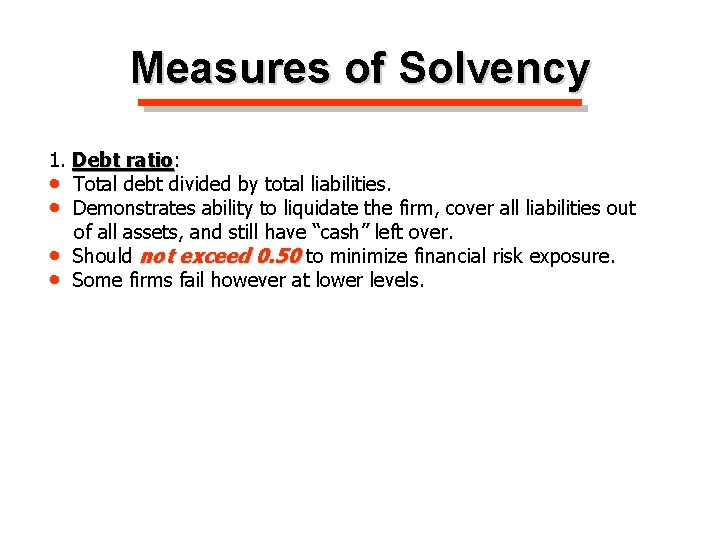Measures of Solvency 1. Debt ratio: ratio • Total debt divided by total liabilities. • Demonstrates ability to liquidate the firm, cover all liabilities out of all assets, and still have “cash” left over. • Should not exceed 0. 50 to minimize financial risk exposure. • Some firms fail however at lower levels.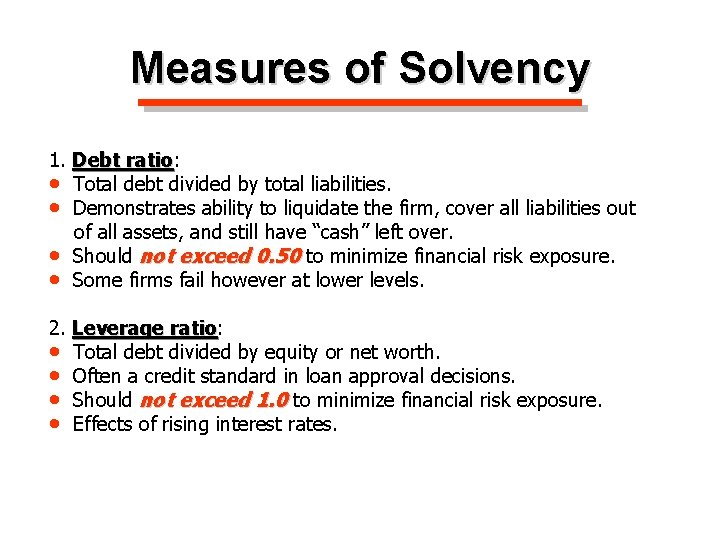Measures of Solvency 1. Debt ratio: ratio • Total debt divided by total liabilities. • Demonstrates ability to liquidate the firm, cover all liabilities out of all assets, and still have “cash” left over. • Should not exceed 0. 50 to minimize financial risk exposure. • Some firms fail however at lower levels. 2. Leverage ratio: ratio • Total debt divided by equity or net worth. • Often a credit standard in loan approval decisions. • Should not exceed 1. 0 to minimize financial risk exposure. • Effects of rising interest rates.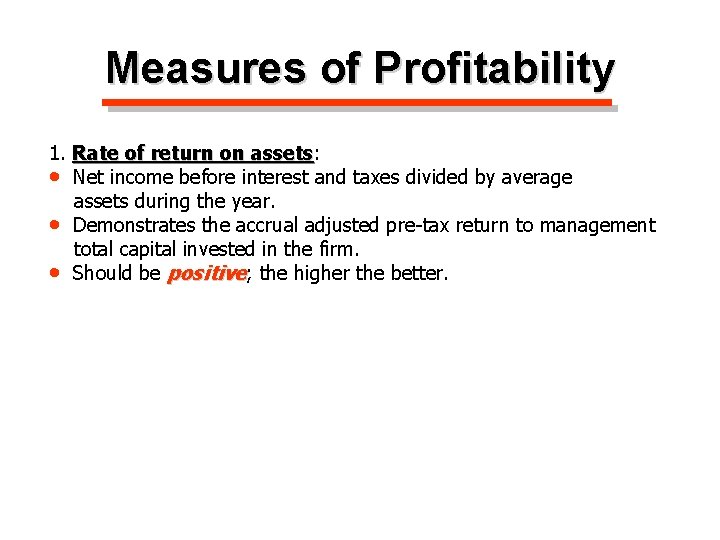Measures of Profitability 1. Rate of return on assets: assets • Net income before interest and taxes divided by average assets during the year. • Demonstrates the accrual adjusted pre-tax return to management total capital invested in the firm. • Should be positive; the higher the better.Measures of Profitability 1. Rate of return on assets: assets • Net income before interest and taxes divided by average assets during the year. • Demonstrates the accrual adjusted pre-tax return to management total capital invested in the firm. • Should be positive; the higher the better. 2. Rate of return on equity: equity • Net income before interest and taxes divided equity. • Demonstrates return on owner equity invested in the firm. • Should be positive; the higher the better.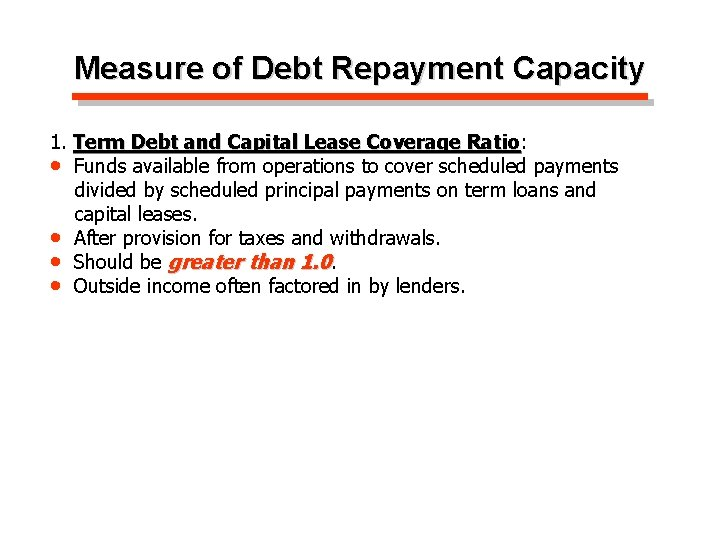Measure of Debt Repayment Capacity 1. Term Debt and Capital Lease Coverage Ratio: Ratio • Funds available from operations to cover scheduled payments divided by scheduled principal payments on term loans and capital leases. • After provision for taxes and withdrawals. • Should be greater than 1. 0. • Outside income often factored in by lenders.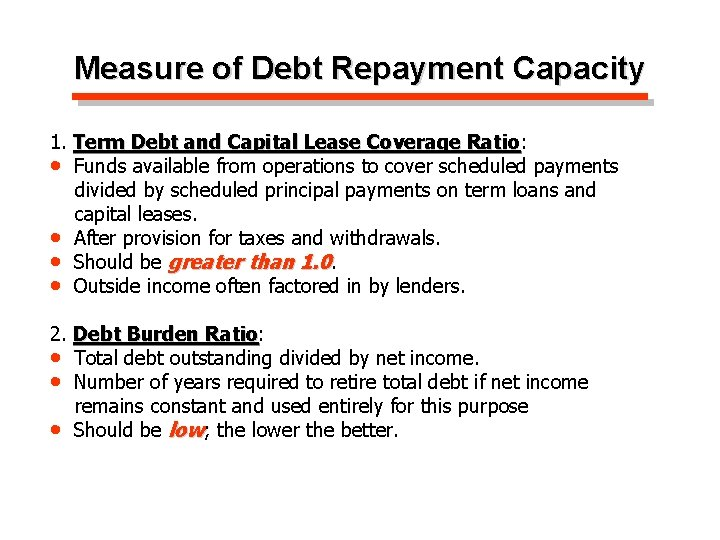Measure of Debt Repayment Capacity 1. Term Debt and Capital Lease Coverage Ratio: Ratio • Funds available from operations to cover scheduled payments divided by scheduled principal payments on term loans and capital leases. • After provision for taxes and withdrawals. • Should be greater than 1. 0. • Outside income often factored in by lenders. 2. Debt Burden Ratio: Ratio • Total debt outstanding divided by net income. • Number of years required to retire total debt if net income remains constant and used entirely for this purpose • Should be low; the lower the better.Know these Concepts üThese are generally accepted financial indicators of a firm’s financial position and strength. üWe will refer to these indicators throughout this course and in MB 664.